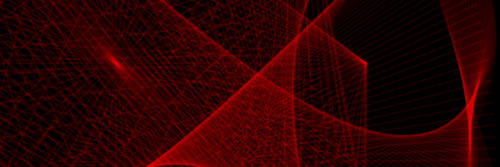# Alba G. Corral

## coder visualist artivist

### a Mathematical ONE

category:

Published:

tags

###### albagcorral ,generative art

by LalodiezOne is the empty product.

One is the smallest positive odd integer.

One is a harmonic divisor number.

One is most often used for representing ‘true’ as a Boolean datatype in computer science.

One is currently considered neither a prime number, nor a composite number — although it used to be considered prime. Defining a prime as a number that is only divisible by one and itself, one is a prime. However, for purposes of factorization and especially the fundamental theorem of arithmetic, it is more convenient to not think of one as a prime factor, or to think of it as an implicit factor that’s always there but need not be written down. To exclude the number one from the list of prime numbers, primality is defined as a number having exactly two distinct positive divisors, one and itself. The last professional mathematician to publicly label 1 a prime number was Henri Lebesgue in 1899. (Carl Sagan included one in a list of prime numbers in his book Contact in 1985.)

One is one of three possible return values of the Möbius function. Passed an integer that is square-free with an even number of distinct prime factors, the Möbius function returns one.

One is the only odd number that is in the range of Euler’s totient function φ(x), in the cases x = 1 and x = 2.

One is the only 1-perfect number (see multiply perfect number).

By definition, 1 is the magnitude or absolute value of a unit vector and a unit matrix (more usually called an identity matrix). Note that the term unit matrix is usually used to mean something quite different.

One is the value of the sine and cosine at π/2 and 0 radians, respectively.

One is the most common leading digit in many sets of data, a consequence of Benford’s law.

Launch ArtWork